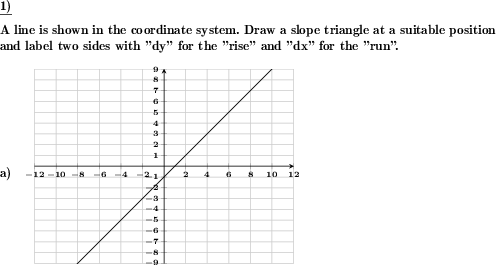Custom math worksheets at your fingertips# Details for problem "Slope triangle to be drawn for a given line"

Quickname: 8003

Elementary School, Primary School, Junior High School, Middle School, High School.

## Summary

In a coordinate system with a given straight line a slope triangle is to be drawn.

## Example## Description

A straight line is given in a coordinate system. Add a slope triangle to this drawing. The task can be extended by the labeling of the gradient triangle, and also by the derivation of the slope.

The straight line equation and the dimensions of the coordinate system are designed in such a way that a gradient triangle can be drawn without any particular difficulty. The intersection of the line and the Y-axis can be chosen to lie on only full or also half coordinate plane squares (vertical divisions).

With respect to the coordinate system, it can be set whether only quadrant one is to be covered with positive X and Y coordinates, or also the quadrants containing coordinates with negative signs. The coordinate system can be plotted in three different sizes.

The slope is chosen randomly, but can also be restricted to be positive or negative. As an additional task a labeling of the gradient triangle can be requested, either with

• "dx"="run" and "dy"="rise" or
• with the actual lengths of dx and dy

can be requested.

If values are required, the derivation of the gradient via the corresponding fraction can also be requested.

Download free printable worksheets for this math problem here. The worksheet contains the problems only, the solution sheet includes the answers. Just click on the respective link.

•Worksheet 1Solution sheet with answers
•Worksheet 2Solution sheet with answers
•Worksheet 3Solution sheet with answers

If you can not see the solution sheets for download, they may be filtered out by an ad blocker that you may have installed. If this is the case, please allow ads for this page and reload the page. The solution sheets will then reappear.

• Do these sample worksheets do not really fit?
• Do you need more math worksheets, with a different level of difficulty?
• Would you like to combine different problems on a worksheet and adjust them to your needs?
• As a teacher, you can put together your own worksheets using the automatically generated math problems provided.
With a free initial credit, you can start creating your own math worksheets in a few minutes.

You can try it for free! Register here, to create custom worksheets now!

## Customization options for this problem

Parameter
Possible values
Number of problems
1, 2
Yes, No
Slope
pos & neg, positive, negative
Sub-square rises
Yes, No
Size
small, medium, large
none, actual lengths, run=dx/rise=dy, derive values,slope

## Similar problems

Remark
Description
Read values only from a provided slope triangle
The slope shall be derived from a straight line with a gradient triangle in the coordinate system.